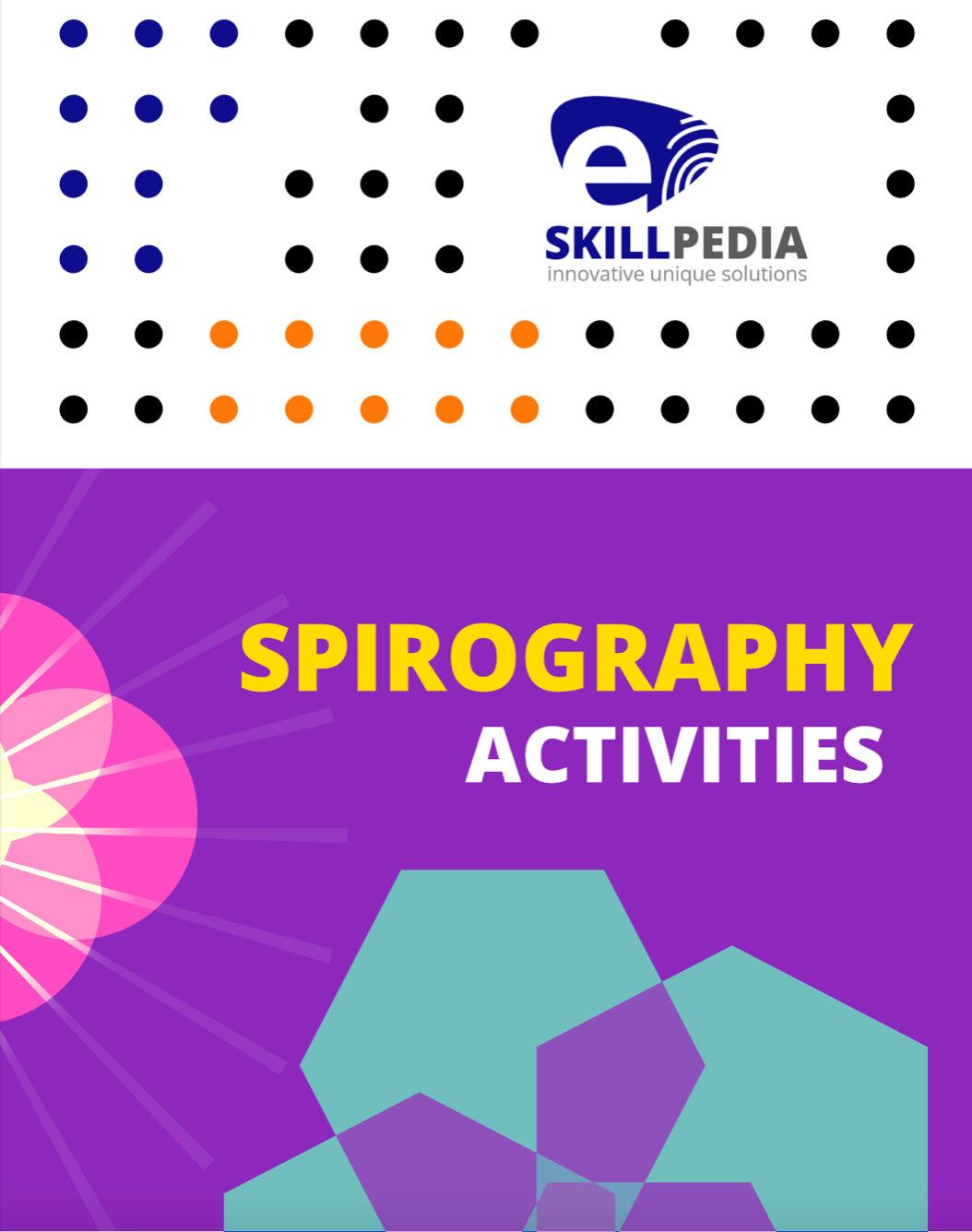### Colouring

Showing 1 to 12 of 41 product(s)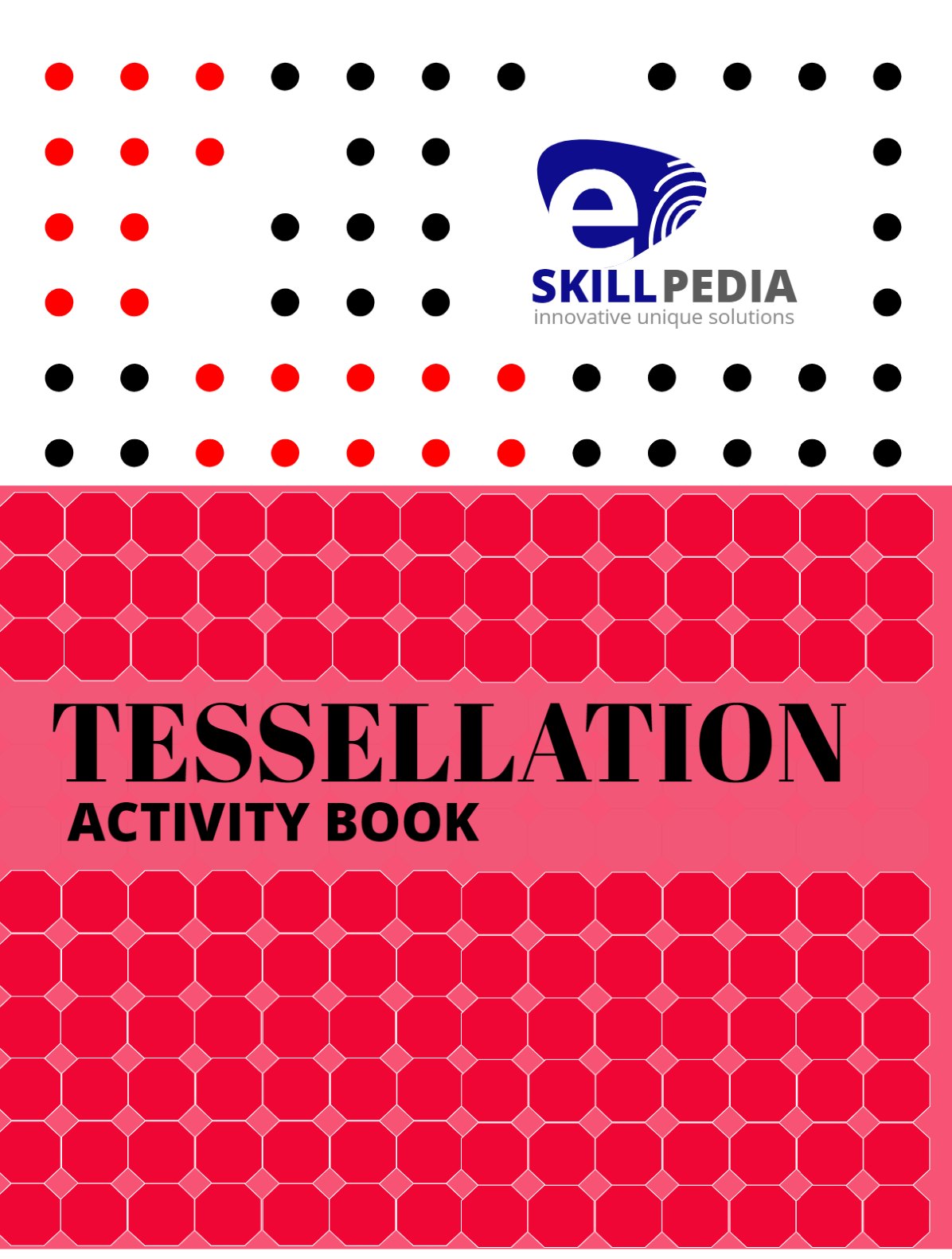### 1. Tessellation Activity Book

A tessellation is the tiling of a plane using one or more geometric shapes such that there are no overlaps

\$2.0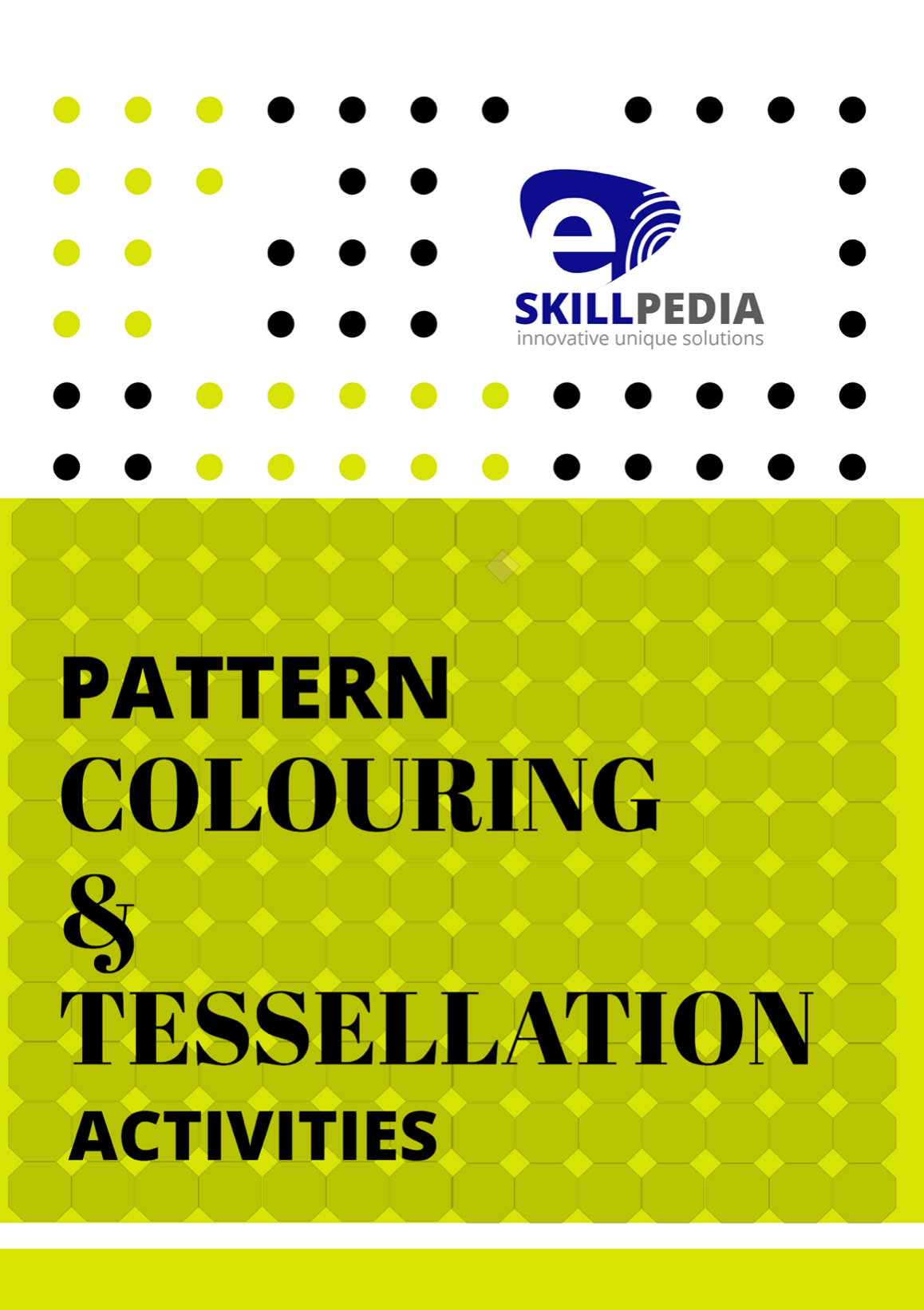### 2. Pattern Colouring & Tessellation Activities

Pattern Colouring Activities:  Pattern colouring is simply a matter of colouring basic shapes, one after

\$2.0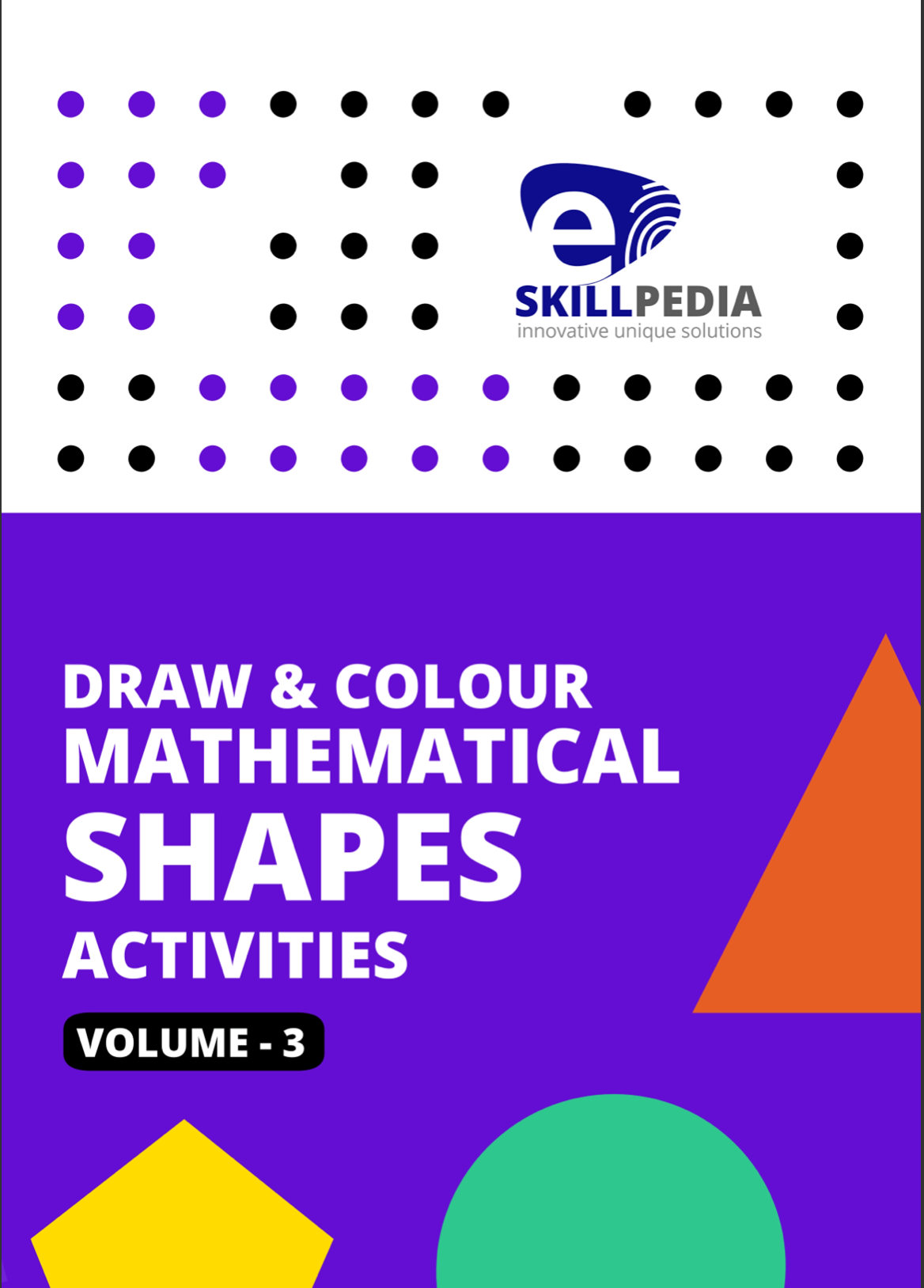### 3. Draw & Colour Mathematical Shapes Activities (Volume 3)

Draw & Colour Mathematical Shapes (Volume 3) This

\$2.0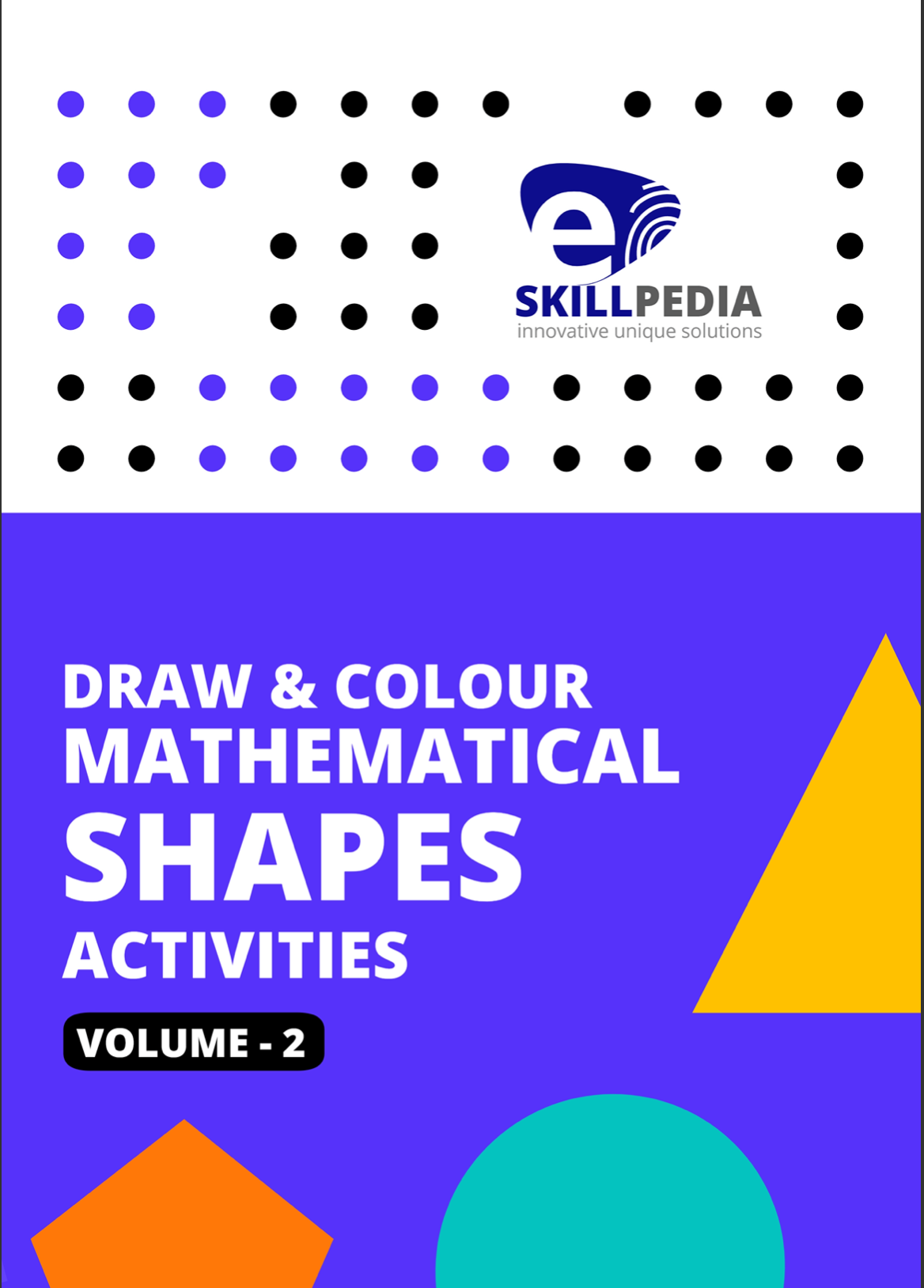### 4. Draw & Colour Mathematical Shapes Activities (Volume 2)

Draw & Colour Mathematical Shapes (Volume - 2) This

\$2.0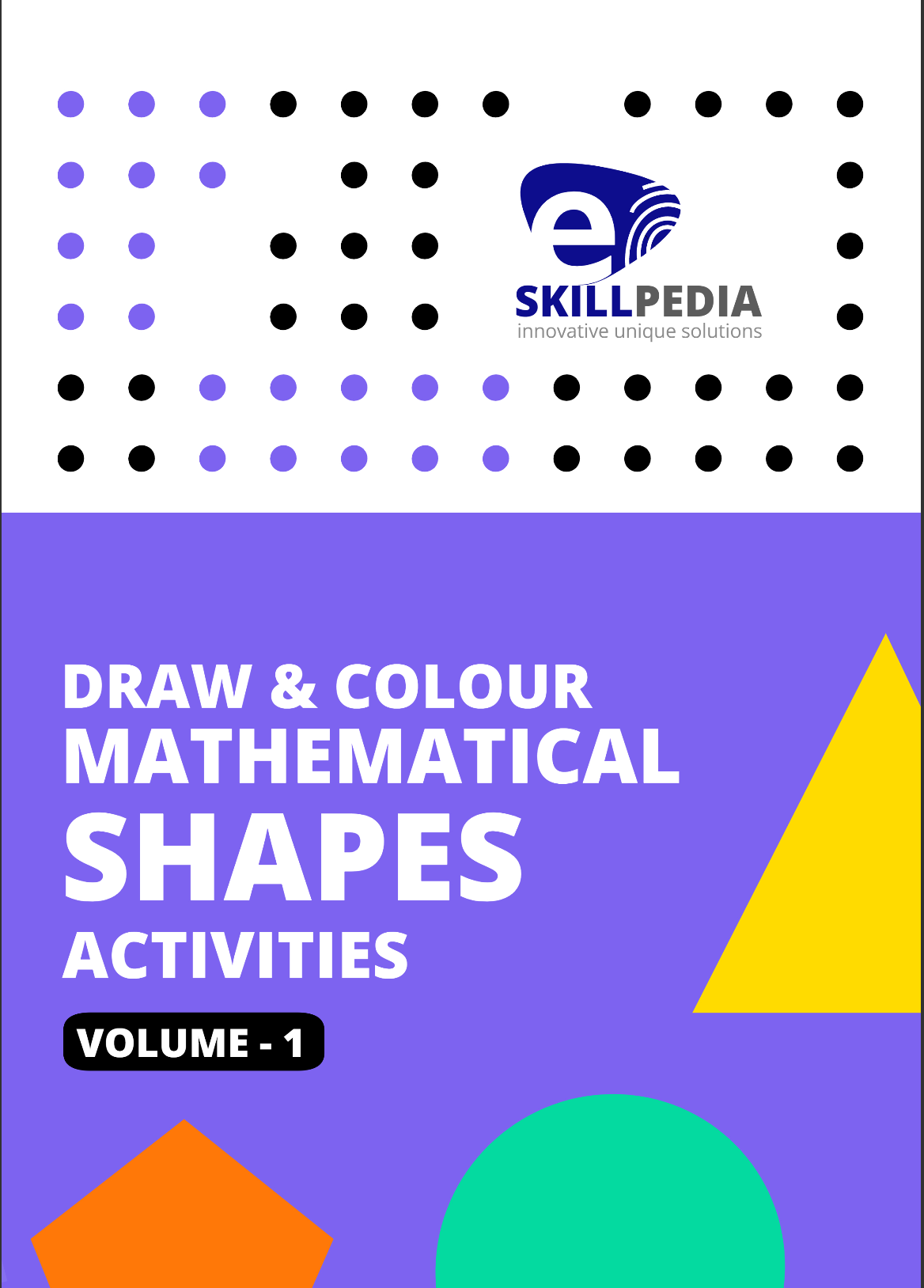### 5. Draw & Colour Mathematical Shapes Activities (Volume - 1)

Draw & Colour Mathematical Shapes (Volume - 1) A